• +91 9971497814
• info@interviewmaterial.com

# Chapter 3- Organisation of Data Interview Questions Answers

### Related Subjects

Question 1 :
The class midpoint is equal to:

(a) The average of the upper class limit and the lower class limit.

(b) The product of upper class limit and the lower class limit.

(c) The ratio of the upper class limit and the lower class limit.

(d) None of the above.

(a) is correct.

The class midpoint is equal to the average of the upper class limit and the lower class limit. It is known by adding the values of upper and lower limits and dividing the total by 2.

Question 2 :
The frequency distribution of two variables is known as

(a) Univariate Distribution

(b) Bivariate Distribution

(c) Multivariate Distribution

(d) None of the above

(b) is correct.

The frequency distribution of two variables is known as Bivariate Frequency Distribution. In other words, Bivariate Frequency Distribution shows the series of statistical data having frequencies of two variables such as the data on income and expenditure of the households.

Question 3 :
Statistical calculations in classified data are based on

(a) the actual values of observations

(b) the upper class limits

(c) the lower class limits

(d) the class midpoints

(d) is correct.

The calculations in classified data or continuous series are based on the class midpoints. The items in a continuous series cannot be exactly measured. Consequently, the class midpoints are calculated.

Question 4 :
Under Exclusive method,

(a) the upper class limit of a class is excluded in the class interval

(b) the upper class limit of a class is included in the class interval

(c) the lower class limit of a class is excluded in the class interval

(d) the lower class limit of a class is included in the class interval

(a) is correct.

A series in which upper limit of one class becomes the lower limit of the succeeding class interval is called exclusive series. In such series, the frequencies of the lower limit are included in that particular class whereas the frequencies of the upper limit are excluded.

Question 5 :
Range is the

(a) difference between the largest and the smallest observations

(b) difference between the smallest and the largest observations

(c) average of the largest and the smallest observations

(d) ratio of the largest to the smallest observation

(a) is correct.

Range is defined as the difference between the largest and the smallest observations.

Algebraically,

R = H – L

Where,

R denotes range

H is the highest value

L is the lowest value

Question 6 :
Can there be any advantage in classifying things? Explain with an example from your daily life.

Yes, there are many advantages of classifying things. The following are the advantages associated with classification:

1. Saves Time and Energy- Classification of things not only saves our time but also our energy which would otherwise be utilised in searching from entire lot of things.

2. Quick Information- Information can be easily collected from the classified things.

3. Easy Classification- Classification facilitates comparisons and helps in drawing fast conclusions or inferences.

The advantage of classification can be better understood with the help of a daily life example. A post office on the regular basis sorts letters and then classifies them according to various attributes. Letters are classified first according to the states, then according to the cities and streets. Thus, this process of classification helps the postman to deliver posts quickly, efficiently and in a non-haphazard manner.

Question 7 :
What is a variable? Distinguish between a discrete and a continuous variable.

A measurable characteristic whose value changes overtime is called variable. It refers to that quantity which keeps on changing and which can be measured by some unit. For example, if we measure the height of students of a class, then height is regarded as a variable. A variable can be either discrete or continuous.
 Discrete Variable Continuous Variable A variable that takes only whole number as its value is called discrete variable. These variables increase in jumps or in complete numbers. For example- Number of people in a family, number of students in a class, etc. A variable that can take any value, within a reasonable limit is called a continuous variable. These variables assume a range of values or increase in fractions and not in jumps. For example- age, height, weight, etc.

Question 8 :
Explain the ‘exclusive’ and ‘inclusive’ methods used in classification of data.

Exclusive Method– This method is used for those series in which the upper limit of one class becomes the lower limit of the next class. It is called as exclusive series because the frequencies of the upper limit of a class interval are not included in that particular class. In such type of series, the upper limit of one class becomes the lower limit of the next class, for example, 0–10, 10–20, 20–30 and so on. The upper limit is excluded but the lower limit is included in the class interval. This method is most appropriate for data of continuous variables.

Inclusive Method– Under this method of classification of data, the classes are formed in such a manner that the upper limit of a class interval does not repeat itself as the lower limit of the next class interval. In such a series, both the upper limit and the lower limit are included in the particular class interval, for example, 1–5, 6–10, 11–15 and so on. The interval 1–5 includes both the limits i.e. 1 and 5.

Question 9 :
Use the data in Table 3.2 that relate to monthly household expenditure (in Rs) on food of 50 households and obtain the range of monthly household expenditure on food.

Calculation of Range

Range = Highest Value – Lowest Value

Highest Value = 5090

Lowest Value = 1007

So, Range = 5090 – 1007 = 4083

Question 10 :
Divide the range into appropriate number of class intervals and obtain the frequency distribution of expenditure.

Preparing Tally Marks

 Class Intervals Tally Marks Frequency 1000 – 1500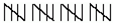20 1500 – 2000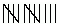13 2000 – 2500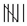06 2500 – 3000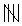05 3000 – 3500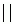02 3500 – 400001 4000 – 450002 4500 – 5000 – 00 5000 – 550001 Total 50

Todays Deals### Chapter 3- Organisation of Data Contributorskrishan

Name:
Email:

# Latest News# 9000 interview questions in different categories